Checkout JEE MAINS 2022 Question Paper Analysis : Checkout JEE MAINS 2022 Question Paper Analysis :

# Invertible Matrices

A matrix is an array of numbers arranged in the form of rows and columns. The number of rows and columns of a matrix are known as its dimensions, which is given by m x n where m and n represent the number of rows and columns respectively. The basic mathematical operations like addition, subtraction, multiplication and division can be done on matrices. In this article, we will discuss the inverse of a matrix or the invertible vertices.

## What is Invertible Matrix?

A matrix A of dimension n x n is called invertible if and only if there exists another matrix B of the same dimension, such that AB = BA = I, where I is the identity matrix of the same order. Matrix B is known as the inverse of matrix A. Inverse of matrix A is symbolically represented by A-1. Invertible matrix is also known as a non-singular matrix or nondegenerate matrix.

For example, matrices A and B are given below: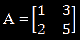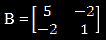Now we multiply A with B and obtain an identity matrix: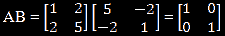Similarly, on multiplying B with A, we obtain the same identity matrix: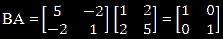It can be concluded here that AB = BA = I. Hence A-1 = B, and B is known as the inverse of A. Similarly, A can also be called an inverse of B, or B-1 = A.

A square matrix that is not invertible is called singular or degenerate. A square matrix is called singular if and only if the value of its determinant is equal to zero. Singular matrices are unique in the sense that if the entries of a square matrix are randomly selected from any finite region on the number line or complex plane, then the probability that the matrix is singular is 0, that means, it will “rarely” be singular.

## Invertible Matrix Theorem

Theorem 1

If there exists an inverse of a square matrix, it is always unique.

Proof:

Let us take A to be a square matrix of order n x n. Let us assume matrices B and C to be inverses of matrix A.

Now AB = BA = I since B is the inverse of matrix A.

Similarly, AC = CA = I.

But, B = BI = B (AC) = (BA) C = IC = C

This proves B = C, or B and C are the same matrices.

Theorem 2:

If A and B are matrices of the same order and are invertible, then (AB)-1 = B-1 A-1.

Proof:

(AB)(AB)-1 = I                                     (From the definition of inverse of a matrix)

A-1 (AB)(AB)-1 = A-1 I                         (Multiplying A-1 on both sides)

(A-1 A) B (AB)-1 = A-1                                   (A-1 I = A-1 )

I B (AB)-1 = A-1

B (AB)-1 = A-1

B-1 B (AB)-1 = B-1 A-1

I (AB)-1 = B-1 A-1

(AB)-1 = B-1 A-1

## Matrix Inversion  Methods

Matrix inversion is the method of finding the other matrix, say B that satisfies the previous equation for the given invertible matrix, say A. Matrix inversion can be found using the following methods:

• Gaussian Elimination
• Newton’s Method
• Cayley-Hamilton Method
• Eigen Decomposition Method

### Applications of Invertible Matrix

For many practical applications, the solution for the system of the equation should be unique and it is necessary that the matrix involved should be invertible. Such applications are:

• Least-squares or Regression
• Simulations
• MIMO Wireless Communications

### Invertible Matrix Example

Now, go through the solved example given below to understand the matrix which can be invertible and how to verify the relationship between matrix inverse and the identity matrix.

Example: If

$$\begin{array}{l}A=\begin{bmatrix} -3 & 1\\ 5 & 0 \end{bmatrix}\end{array}$$
and
$$\begin{array}{l}B=\begin{bmatrix} 0 & \frac{1}{5}\\ 1 & \frac{3}{5} \end{bmatrix}\end{array}$$
, then show that A is invertible matrix and B is its inverse.

Solution:

Given,

$$\begin{array}{l}A=\begin{bmatrix} -3 & 1\\ 5 & 0 \end{bmatrix}\end{array}$$
and
$$\begin{array}{l}B=\begin{bmatrix} 0 & \frac{1}{5}\\ 1 & \frac{3}{5} \end{bmatrix}\end{array}$$

Now, finding the determinant of A,

$$\begin{array}{l}|A|=\begin{vmatrix} -3 & 1\\ 5 & 0 \end{vmatrix}\end{array}$$

= -3(0) – 1(5)

= 0 – 5

= -5 ≠ 0

Thus, A is an invertible matrix.

We know that, if A is invertible and B is its inverse, then AB = BA = I, where I is an identity matrix.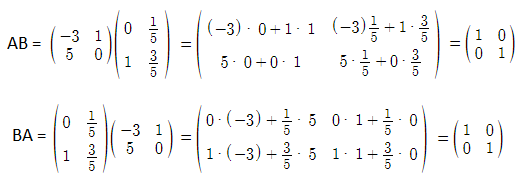AB = BA = I

Therefore, the matrix A is invertible and the matrix B is its inverse.

### Properties

Below are the following properties hold for an invertible matrix A:

• (A−1)−1 = A
• (kA)−1 = k−1A−1 for any nonzero scalar k
• (Ax)+ = x+A−1 if A has orthonormal columns, where + denotes the Moore–Penrose inverse and x is a vector
• (AT)−1 = (A−1)T
• For any invertible n x n matrices A and B, (AB)−1 = B−1A−1. More specifically, if A1, A2…, Ak are invertible n x n matrices, then (A1A2⋅⋅⋅Ak-1Ak)−1 = A−1kA−1k−1⋯A−12A−11
• det A−1 = (det A)−1